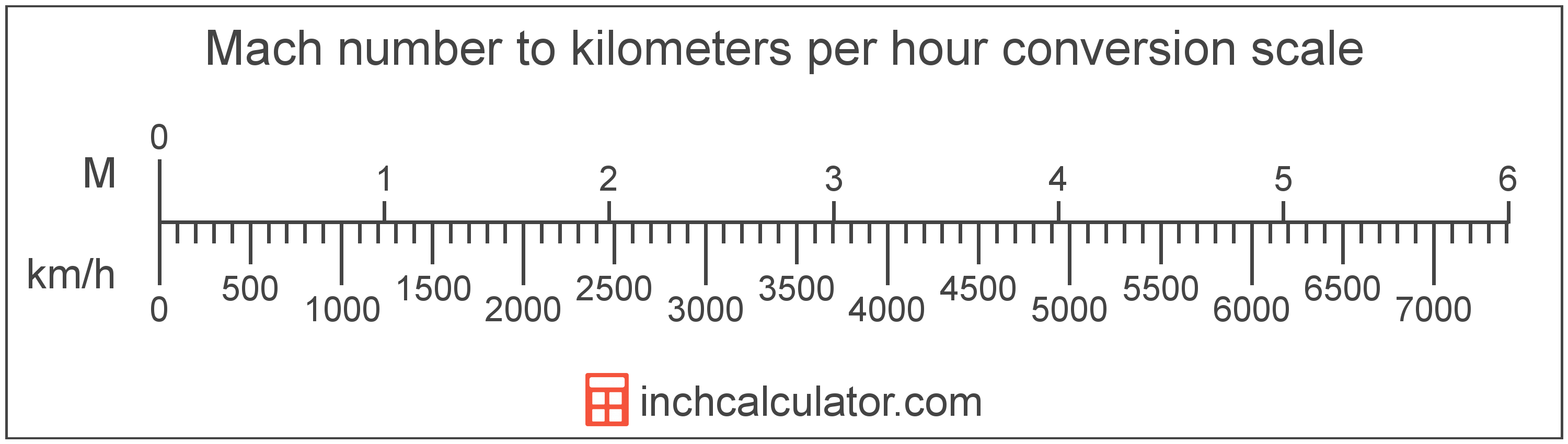# Mach Number to Kilometers Per Hour Conversion

Enter the Mach number below to convert the speed to kilometers per hour.

Results in Kilometers Per Hour:Mach 1 = 1,234.8 km/h

## How to Convert Mach Number to Kilometers Per HourTo convert a Mach measurement to a kilometer per hour measurement, multiply the Mach number by the conversion ratio. Mach 1 is equal to 1,234.8 kilometers per hour, so use this simple formula to convert:

kilometers per hour = Mach number × 1,234.8

The speed in kilometers per hour is equal to the Mach number multiplied by 1,234.8.

For example, here's how to convert Mach 2 to kilometers per hour using the formula above.
Mach 2 = (2 × 1,234.8) = 2,469.6 km/h

Mach number and kilometers per hour are both units used to measure speed. Keep reading to learn more about each unit of measure.

## Mach Number

Mach, sometimes also referred to as a Mach number, is the ratio of the speed of an object to the speed of sound.

Mach can be abbreviated as M, and is also sometimes abbreviated as Ma. For example, Mach 1 can be written as 1 M or 1 Ma.

Although Mach can be abbreviated M or Ma, the Mach number is typically expressed with the number after the unit name. For example, the expression Mach 3 is preferred rather than 3 Machs or 3 M.

The speed is classified as either subsonic, transonic, supersonic, or hypersonic depending on the Mach number.

Speed classifications for various mach numbers.
Speed Classification Mach Number
Subsonic Mach < 1.0
Transonic Mach = 1.0
Supersonic Mach > 1.0
Hypersonic Mach > 5.0

## Kilometers Per Hour

Kilometers per hour are a measurement of speed expressing the distance traveled in kilometers in one hour.

The kilometer per hour, or kilometre per hour, is an SI unit of speed in the metric system. Kilometers per hour can be abbreviated as km/h, and are also sometimes abbreviated as kph. For example, 1 kilometer per hour can be written as 1 km/h or 1 kph.

In formal expressions, the slash, or solidus (/), is used to separate units used to indicate division in an expression.

Kilometers per hour can be expressed using the formula:
vkm/h = dkmthr

The velocity in kilometers per hour is equal to the distance in kilometers divided by time in hours.

## Mach to Kilometer Per Hour Conversion Table

Mach numbers converted to kilometers per hour
Mach Number Kilometers Per Hour
Mach 0.25 308.7 km/h
Mach 0.5 617.4 km/h
Mach 0.75 926.1 km/h
Mach 1 1,235 km/h
Mach 1.5 1,852 km/h
Mach 2 2,470 km/h
Mach 2.5 3,087 km/h
Mach 3 3,704 km/h
Mach 3.5 4,322 km/h
Mach 4 4,939 km/h
Mach 4.5 5,557 km/h
Mach 5 6,174 km/h
Mach 5.5 6,791 km/h
Mach 6 7,409 km/h
Mach 6.5 8,026 km/h
Mach 7 8,644 km/h
Mach 7.5 9,261 km/h
Mach 8 9,878 km/h
Mach 8.5 10,496 km/h
Mach 9 11,113 km/h
Mach 9.5 11,731 km/h
Mach 10 12,348 km/h

## References

1. NASA, Mach Number, https://www.grc.nasa.gov/WWW/BGH/mach.html
2. National Institute of Standards and Technology, NIST Guide to the SI, Chapter 6: Rules and Style Conventions for Printing and Using Units, https://www.nist.gov/pml/special-publication-811/nist-guide-si-chapter-6-rules-and-style-conventions-printing-and-using